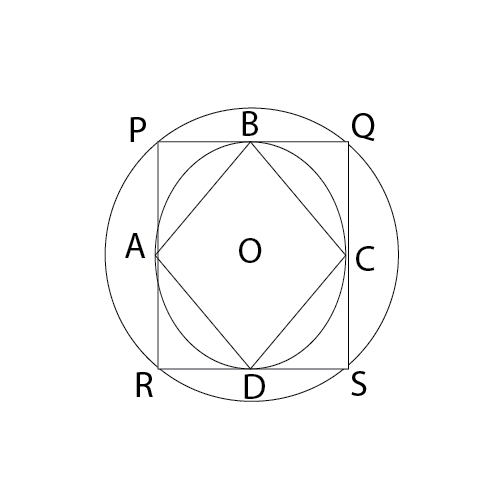Question 13

# The figure below shows two concentric circles with centre 0. PQRS is a square, inscribed in the outer circle. It also circumscribes the inner circle, touching it at points B, C, D and A. What is the ratio of the perimeter of the outer circle to that of polygon ABCD?Solution

By symmetry, it is safe to assume that the polygon ABCD is a square. So, AB = PO. The perimeter of the inner square = 4 AB. The perimeter of the outer circle = $$2 \pi \times AB$$

So, ratio = $$\frac{2 \pi \times AB}{4AB}$$ = $$\frac{\pi}{2}$$